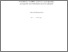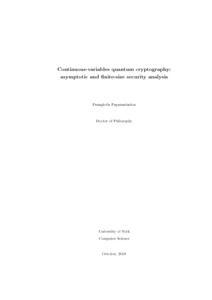# Continuous-variables quantum cryptography: asymptotic and finite-size security analysis

Papanastasiou, Panagiotis (2018) Continuous-variables quantum cryptography: asymptotic and finite-size security analysis. PhD thesis, University of York.Preview
Text
thesis3.pdf - Examined Thesis (PDF)

## Abstract

In this thesis we study the finite-size analysis of two continuous-variables quantum key distribution schemes. The first one is the one-way protocol using Gaussian modulation of thermal states and the other is the measurement- device-independent protocol. To do so, we adopt an efficient channel parameter estimation method based on the assumption of the Gaussian variables and the central limit theorem introduced by Ruppert et al. [Phys. Rev. A 90, 062310 (2014)]. Furthermore, we present a composable security analysis of the measurement device independent protocol for coherent attacks with a channel parameter estimation that is not based on the central limit theorem. We also investigated, in the asymptotic regime, an asymmetric situation for the authenticated parties against the eavesdropper caused by fast-fading channels. Here we assume that the eavesdropper has the full control of the communication channel and can instantaneously change its transmissivity in every use of it. We assumed the simple model of a uniform fading and addressed the cases of one-way protocols, continuous-measurement-device- independent protocol in symmetric configuration and its star network extension for three users. Finally, we extended the asymptotic study of the one-way protocols using an arbitrary number of phase-encoded coherent states assuming a thermal loss channel without using a Gaussian approximation.

Item Type: Thesis (PhD) thermal-state QKD, CV-MDI-QKD, discrete modulation CV-QKD The University of York > Computer Science (York) uk.bl.ethos.778875 Mr Panagiotis Papanastasiou 04 Jun 2019 13:45 19 Feb 2020 13:08 http://etheses.whiterose.ac.uk/id/eprint/23774

### Available Versions of this Item

• Continuous-variables quantum cryptography: asymptotic and finite-size security analysis. (deposited 04 Jun 2019 13:45) [Currently Displayed]